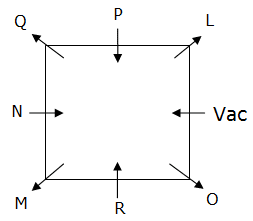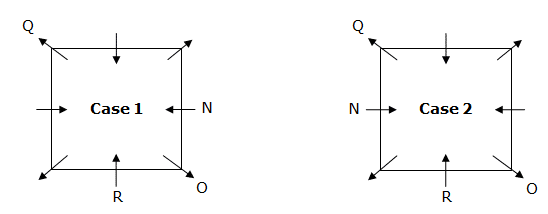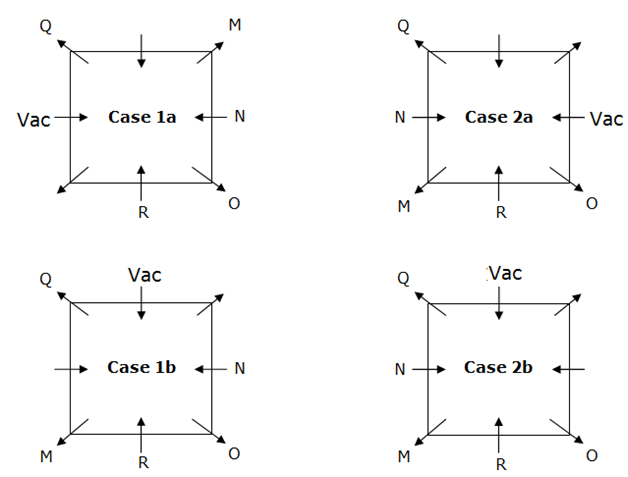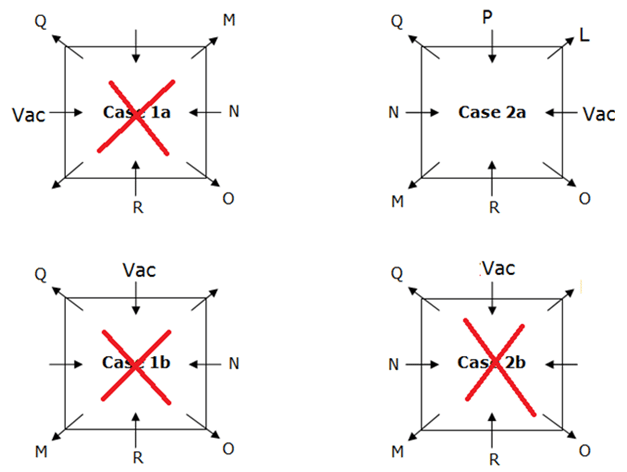# SBI Clerk Prelims Reasoning Ability Questions 2020 (Day-09)

Dear Aspirants, Our IBPS Guide team is providing new series of Reasoning Questions for SBI Clerk Prelims 2020 so the aspirants can practice it on a daily basis. These questions are framed by our skilled experts after understanding your needs thoroughly. Aspirants can practice these new series questions daily to familiarize with the exact exam pattern and make your preparation effective.

[WpProQuiz 7634]

Ensure Your Ability Before the Exam – Take SBI Clerk 2020 Prelims Free Mock Test

Seating arrangement

Directions (1-5): Study the following information carefully and answer the questions given below.

A square table consists of 8 seats. 7 Persons L, M, N, O, P, Q, R are seated around the square table such that the persons sitting at the corners faces away from the center while the persons sitting at the middle of the sides faces towards the center,  but not necessarily in the same order. R sits third to the left of Q, who sits at one of the corners of the table. Only one person sits between N and R. O sits at the immediate right of R. Two persons sit between M and the vacant seat, which is at one of the sides of the square table. L, who sits at the immediate left of P and is an immediate neighbor of the vacant seat.

1) How many persons sit between L and R, when counted from the right of L?

a) None

b) One

c) Two

d) Three

e) More than three

2) What is the position of N with respect to O?

a) Third to the left

b) Second to the right

c) Immediate left

d) Third to the right

e) Second to the left

3) Four of the following five are alike in a certain way and hence forms a group. Which of the following does not belong to the group?

a) Q

b) M

c) R

d) L

e) O

4) Which of the following pair represents the persons sitting immediately next to the vacant seat?

a) L, O

b) O, M

c) Q, L

d) P, O

e) Q, P

5) Who sits at the immediate left of R?

a) L

b) P

c) N

d) M

e) Q

Inequality

Directions (6-8): In each of the following questions, relationship between different elements is shown in the statements followed by two conclusions. Find the conclusion which is definitely true.

a) If only conclusion I follows.

b) If only conclusion II follows.

c) If either conclusion I or II follows.

d) If neither conclusion I nor II follows.

e) If both conclusions I and II follow.

6) Statements:

A ≤ B > C = D ≥ E > F

Conclusions:

I) E ≤ C

II) A < C

7) Statements:

G > H = I ≥ J = K ≤ L

Conclusions:

I) J < L

II) L = J

8) Statements:

M = N < O ≤ P ≥ Q = R

Conclusions:

I) P ≥ R

II) M < P

Order and Ranking

Directions (9-10): Study the following information carefully and answer the questions given below.

Six boxes A, B, C, D, E and F contains different number of boxes. The boxes are arranged according to the number of chocolates from highest to lowest. Box E contains lesser chocolates than Box A but more chocolates than Box B, which is not the box with lowest number of chocolates. Box F has less number of chocolates than B. Box C contains lesser chocolates than Box D, which has the highest number of chocolates but more than Box E.

9) Which box contains the lowest number of chocolates?

a) Box B

b) Box C

c) Box A

d) Box F

e) Box E

10) If Box B contains 100 chocolates, then how many chocolates does Box F have?

a) 77 chocolates

b) 153 chocolates

c) 165 chocolates

d) 139 chocolates

e) 175 chocolates

Directions (1-5):R sits third to the left of Q, who sits at one of the corners of the table. Only one person sits between N and R. O sits at the immediate right of R.Two persons sit between M and the vacant seat, which is at one of the sides of the square.L, who sits at the immediate left of P is an immediate neighbor of the vacant seat.Since L cannot be placed, Case 1a, Case 1b gets cancelled.

Case 2b gets cancelled because L, who sits at the immediate left of P.

Hence Case 2a will be the final arrangement.

Directions (6-8):

I) E ≤ C (C = D ≥ E) -> True

II) A < C (A ≤ B > C) -> False

So, only conclusion I follow.

I) J < L (J = K ≤ L)

II) L = J (J = K ≤ L)

So, either conclusion I or II follows.

I) P ≥ R (P ≥ Q = R) -> True

II) M < P (M = N < O ≤ P) -> True

So, both conclusion I and II follow.